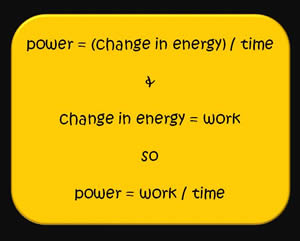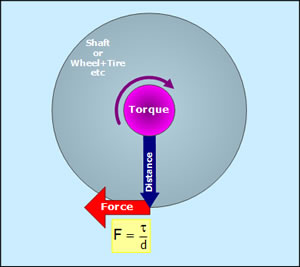# Difference between Power and Torque

##### Key Difference: Power and torque are two important terms used in physics. Power defines the rate of doing a work, whereas torque represents the measure of energy that is applied while rotating an object about an axis or pivot point. Power is a scalar quantity, whereas torque is a vector quantity.

Power and torque may seem to be difficult technical terms, but on the contrary they are interesting and we also apply them in our day to day life. Let us find out the differences between them.You apply a force to climb the stairs. It may be a regular task but in physics it is termed as the work as it involves a force and a movement in the direction of the force. For example, a force of 10 Newton pushing an object 2 meters in the direction of force is equivalent to (10 *2) 20 joules of work.

You may think that what was the need of explaining work? It is important as the definition of power includes the term work. Power is defined as the rate of doing work or rate of using energy. For example, if you do 75 Joules of work in one second then the power is simply 75 Watts.The SI unit of power is the watt (W) or joule per second (J/s). Power defines how fast or quickly energy is being used. Power can also be described as the force multiplied by the velocity of an object. You may also come across the word horsepower and must have observed it many times in the description of vehicles. It defines the power of an engine. It is equivalent to the power that is needed to move 33,000 pounds to one foot and in one second.  Power is a scalar quantity which means that it has no relation to the direction.

Torque is also known as the moment or moment of force; it is the measure of force that rotates an object about its axis, fulcrum or pivot. It can simply be considered as a twist to an object. For example, when you simply turn the knob of your door, then torque is being used.

Torque is a vector quantity which means that it has both magnitude as well as the direction. Since it involves the rotation of an object, the object can rotate in either clockwise direction or anti-clockwise direction. It is generally represented with the symbol τ. It is defined as the = r x F = r F sin(a), where r is the distance from the pivot point to the point where force is applied and F is the force applied, whereas a is the angle between r and f. The right hand rule is applied to determine the direction of the torque.

Thus, we can say that both are different from each other. Power defines the rate at which work is done. On the other hand, torque is the measure of force applied to rotate an object about an axis. The unit of power is joules per second, whereas torque is defined as Newton-meter or joules per radian.  Power is related to movement of an object, whereas torque can be generated without moving an object.

Image Courtesy: msclantonsphysicalsciencepage.weebly, craig.backfire.ca